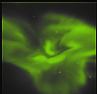Next: Summary Up: Tomography Previous: Error sensitivity   Contents

# Stopping criteria

It has long been known that iterative solutions to tomographic problems improve initially but deteriorate after some point ( Gordon and Herman, 1974). This highlights the importance of knowing when it is time to stop.

What we want to do is to test whether the reconstruction gives projections that are statistically compatible with the observed images. If we had a large number of samples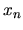from one random process, it is possible to test whether the random process has a hypothetical probability distribution. The method to do this is the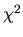-test of fit for which the range of the random variable is divided into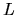intervals,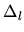with theoretical probabilities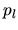for a random sample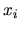to fall into the interval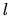if the hypothesis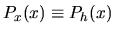is true.

With a total of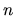samples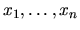andsamples in interval, the-test function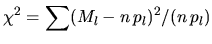(3.15)

has an asymptotic-distribution with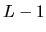degrees of freedom.is a measure of the deviation between the true distribution and the hypothetical one. The hypothesis is accepted if the value calculated from equation (3.15) is less than a critical value corresponding to a chosen significance level ( Bronstein and Semendyayev, 1997).

In the case of tomography, the pixels in the projection images of the reconstructed three-dimensional source distribution are the expected values of the probability distributions from which the measured pixel values are the random samples. Here we only have one sample from each probability distribution; it should be noted that the probability distribution for a pixel value is known and assumed to be determined only from the expected value.

For auroral imaging with ALIS, the pixel intensity has a probability distribution that is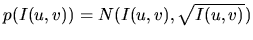as derived in section 3.1. With the above relation between expected pixel intensity and the random distribution of the measured pixel intensity, it is possible to use the above-test after the modification that the random process for each pixel is divided into intervals with equal probabilitiesand that the measured pixel intensity is put into its corresponding interval.

The hypothesis that the measured images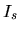are random samples from a set of random processes with expected values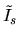is accepted if thevalue calculated from equation (3.15) is less than is required at the corresponding significance level. (The values ofare calculated from the source distribution.) For processes that have continuous probability distributions, it is no problem to divide the range into equal intervals for different expected values and widths but for discrete probability distributions this can be a problem; for Poissonian processes a method to overcome this problem is described by Veklerov and Llacer (1987).

An example that can illustrate the working procedure outlined above is to look at the outcome of the roll of four non-standard dice. What separates these dice from ordinary dice is that they have unknown numbers of dots; that is, they might have dots from 1 to 6 or from 3 to 8 or any other sequence of dots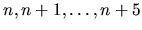. If we make a measurement of the dot numbers and then get two models which estimate the expected value of each die, as presented in table 3.4, the algorithm for this stopping criteria is as follows.First we divide the probability distribution into a number of intervals, for this case two intervalswith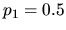and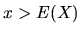with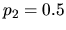. For model 1 all events fall into interval 1 and thevalue according to equation (3.15) is:(3.16)

and for model 2 diefalls into interval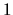and dice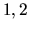and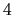fall into interval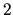giving avalue, according to equation (3.15), of: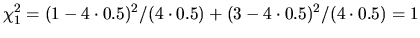(3.17)

For a significance level of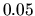and one degree of freedom, the critical value ofis 3.84, i.e. ifis larger than 3.84 we must reject the hypothesis that the model is the cause of the observations. Model 1, with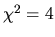, is not likely given the observations and has to be rejected, and for model 2, with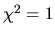, we cannot reject the hypothesis and should be satisfied and stop the iteration.

If we have come this far the reconstruction gives projections that fit the measured images in a statistical sense. However, there are not yet any guarantees that the spatial distribution of the errors is well behaved, i.e., the large positive errors might be grouped together in one region of the images and small errors might be grouped in another region. One further condition for optimal reconstructions is that the spatial distribution of the errors is also random. This can be tested with the same method as above: the image location of errors in an intensity intervalis calculated and then the images are divided into 10 by 10 regions and the number of errors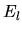from the intervalis calculated region by region. If the-test function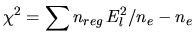(3.18)

where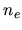is the total number of errors in the intensity error intervaland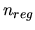is the total number of regions, is also less than the corresponding significance value, there is no reason to continue with the iteration.

By the way, about the cartoon animation on the even pages - it starts of at page 2. The images in the top right corner are ALIS data of HF enhanced airglow from Silkimuotka and the images in the lower left corner is the projected images of the retrieved volume emission. The animation starts at 17:40:15 UT with 10 s between each frame and covers about one HF-pump on-off cycle.Next: Summary Up: Tomography Previous: Error sensitivity   Contents

copyright Björn Gustavsson 2000-10-24